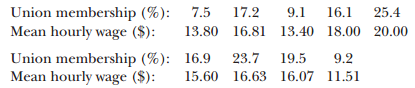Create an Account

Home / Questions / The percentages of workers in trade unions and the mean hourly wage in nine US states are ...

The percentages of workers in trade unions and the mean hourly wage in nine US states are a Which one of these should be the dependent variable Y b Plot the data on a scatter diagram

The percentages of workers in trade unions and the mean hourly wage in nine US states are:(a) Which one of these should be the dependent variable (Y)?

(b) Plot the data on a scatter diagram.

(c) Determine the equation of the line of best fit and plot it on your diagram.

(d) Find the coefficient of determination and assess its value.

Jul 23 2020 View more View LessSubscribe To Get Solution